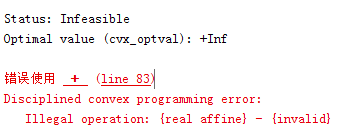# Illegal operation: {real affine} - {invalid} in CVX

Dear everyone,
The following constraints

`R<=sum_square(a_l+x_l)+sum(2*(a_l+x_l).*(a-a_l))+sum(2*(a_l+x_l).*(x-x_l))-(sum_square(x)+sum_square(a));`

where ,R is a constant `1*K` vector , a_l and x_l are the constant `N*K` real matrix, the x and a are `N*K` variable matrix .
The CVX results show failure as following,